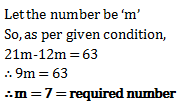# Problems on Numbers - Quantitative Aptitude (MCQ) questions for Q. 29130

Q.  A boy was asked to multiply a number by 12. But he multiplied by 21 and got his answer 63 more than the correct answer. The number to be multiplied was
- Published on 05 Apr 17

a. 7
b. 9
c. 8
d. 12